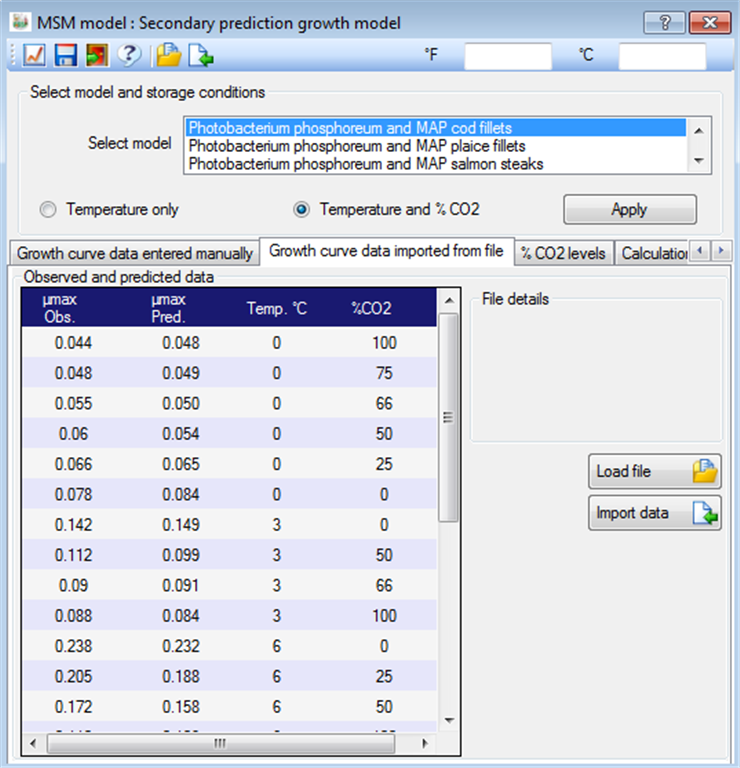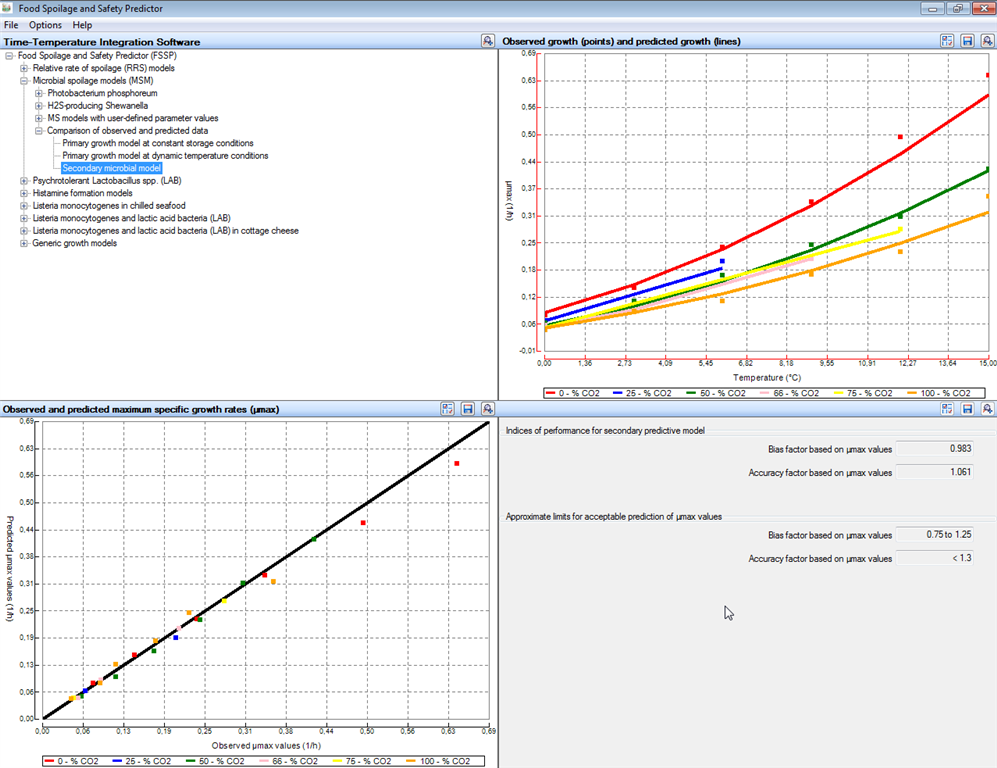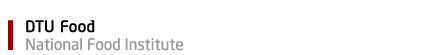Module Microbial spoilage models:  Comparison of observed and predicted data
 This module allows data for growth of Photobacterium phosphoreum and H2S-producing Shewanella bacteria to be compared with prediction produced by FSSP. The module has been divided into three sub-modules to handle different types of data: Primary growth model at constant storage conditions (Change in concentration of bacteria with time) Primary growth model at dynamic temperature conditions (Change in concentration of bacteria with time) Secondary microbial model (Effect of storage conditions on the growth rate of bacteria)
 Primary growth model at constant storage conditions [FSSP home]Firstly, a microbial spoilage model and appropriate storage conditions must be selected in FSSP to generate the predicted growth. Then data for observed growth of the corresponding bacterium and storage condition can be entered manually or read from ASCII and XML files (See FSSP dialog box below).By clicking on the graph icon (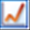upper left corner in dialog box above) the software compares the observed and predicted growth as shown in the two FSSP output windows below. In the first of the two output windows the observed and predicted growth is similar as shown both by the comparison graph and by the three indices of model performance i.e. (i) the root mean square error (Eqn 1), (ii) the modified bias factor based on log(cfu/g) (Eqn 2) and (iii) the modified accuracy factor based on log(cfu/g) (Eqn 3). In the second output windows below the observed growth is faster than the predicted growth. As shown in that FSSP output window this results in (i) an increased root mean square error, (ii) a bias factor below 1 and (iii) an accuracy factor well above 1.Eqn. 1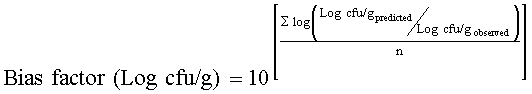Eqn. 2Eqn. 3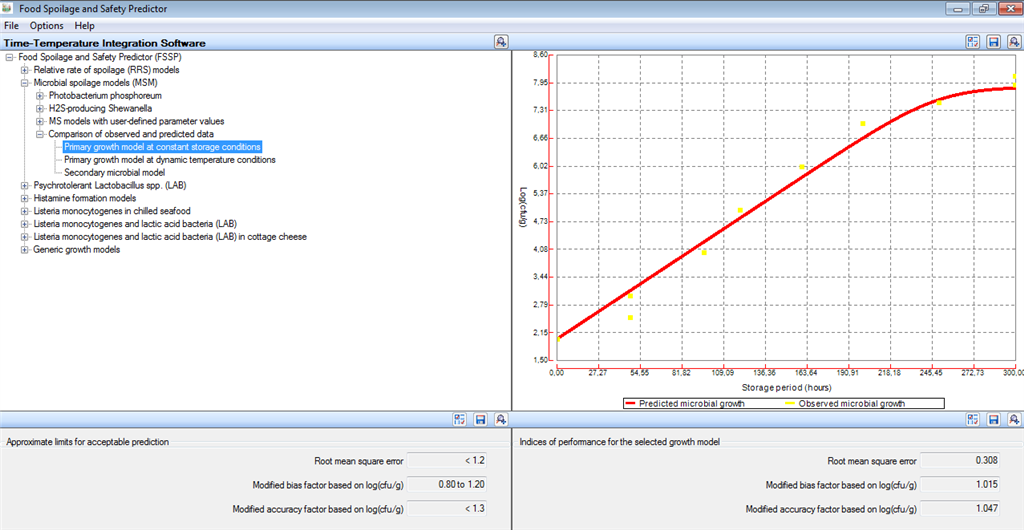Primary growth model at dynamic temperature conditions [FSSP home] This FSSP module function in the same way as the module above for Primary growth model at constant storage conditions except that temperature profile data must be imported as ASCII- or XML-files (See dialog box below). The FSSP output window shows (i) the evaluated temperature profile, (ii) observed and predicted growth as well as (iii) indices of model performance.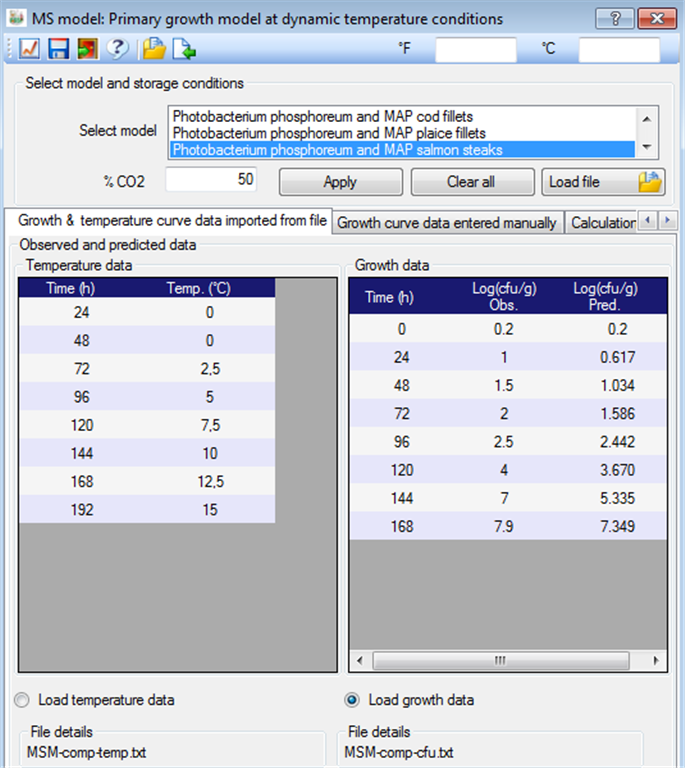Secondary microbial growth models [FSSP home] This module function in the same way as the module Primary growth model at constant storage conditions. However, the comparison of observed and predicted data rely on values of maximum specific growth rates (µmax) rather than the concentration of bacteria (Log cfu/g) as used with the primary growth models. Growth rate data must be entered manually or read from an ASCII or XML-file (See dialog box below). FSSP then produces comparison graphs and calculates indices of model performance i.e. (i) the bias factor (Eqn 4) and (ii) the accuracy factor (Eqn 5). The FSSP output window below shows an example with very good agreement between observed and predicted µmax-values.Eqn. 4Eqn. 5